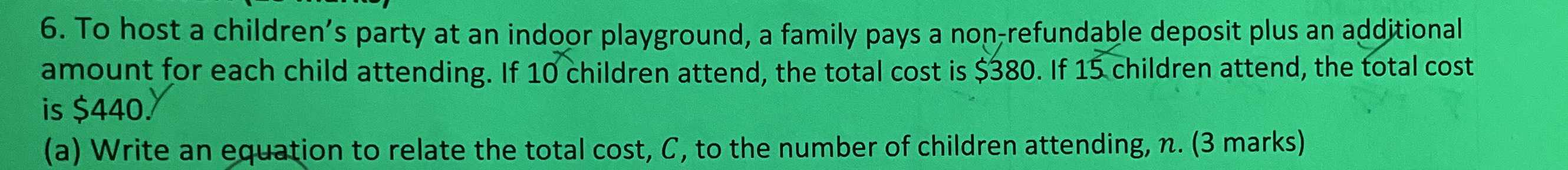### ¿Todavía tienes preguntas de matemáticas?

Pregunte a nuestros tutores expertos
Algebra
Pregunta6. To host a children's party at an indoor playground, a family pays a non-refundable deposit plus an additional amount for each child attending. If $$10$$ children attend, the total cost is $$\ 380$$ . If $$15$$ children attend, the total cost is $$\ 440$$ .

(a) Write an equation to relate the total cost, $$C$$ , to the number of children attending, $$n . ( 3$$ marks)